# Empty value as a result of an if then else calculation

I have a have a simple calculation: "if sum(0, 0, m1) <= 0 then -1 else sum(0, 0, m1)"

Instead of the value "-1" I would like to have the value NULL (empty). Is it possible to do that?

• Hi Flemming,

I have an idea related to number formatting. Not sure it will work in your case, but here it is.

First, you should change your calculation to this:

if sum(0, 0, m1) <= 0 then 0 else sum(0, 0, m1)

Secondly, you can work with Number formatting, where zero numbers can be set to no digits. With no digits, it will turn up as a blank instead of '0':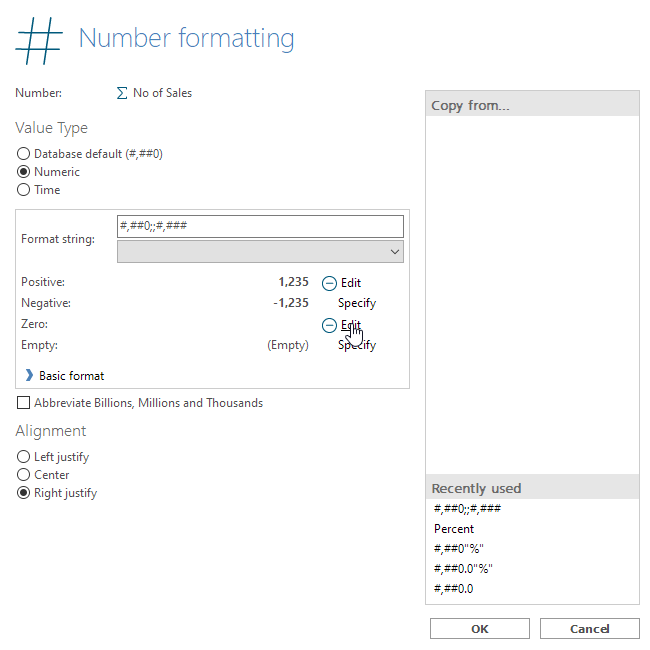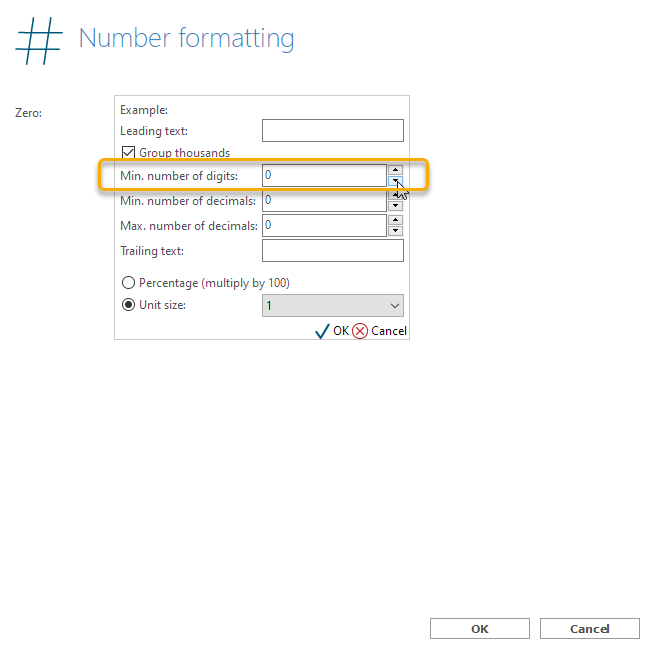BR / Ole

• I think text like "NULL" is not possible in a measure column.

But instead you could make your calculation and after that you can hide the value with the visibility agent.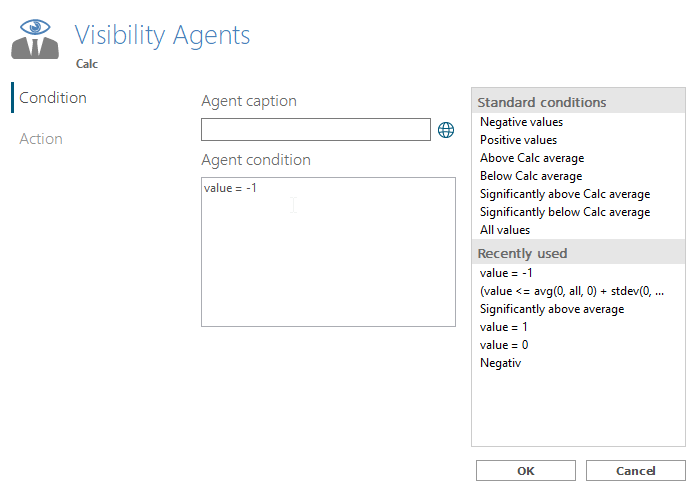• Thanks - visibility agents can only partly solve my problem (a complex analysis ;-))

I don't need a text like "NULL" - I need the measure to be empty like marked below, when the value is O or less: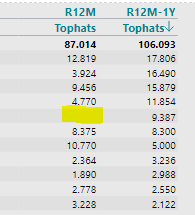• I would have suggested a visibility agent as well :-)

Can you explain why a visibility agent with syntax <= 0 can't do the job?

• Hi Ole

It did some of the work.

But I have a calulation counting the number of Customers (with a turnover above 0) and this calculation still count Customers with the hidden zero value (the first column R12M only have 28 customers but the total of buying customers is 29)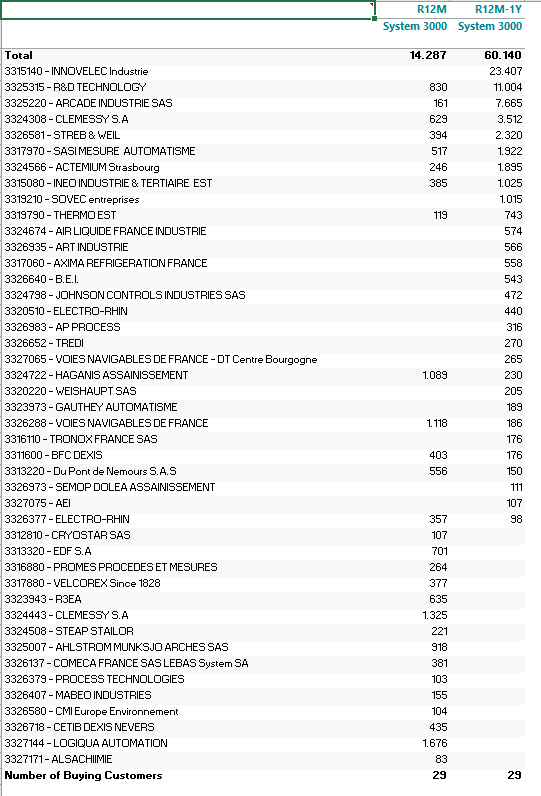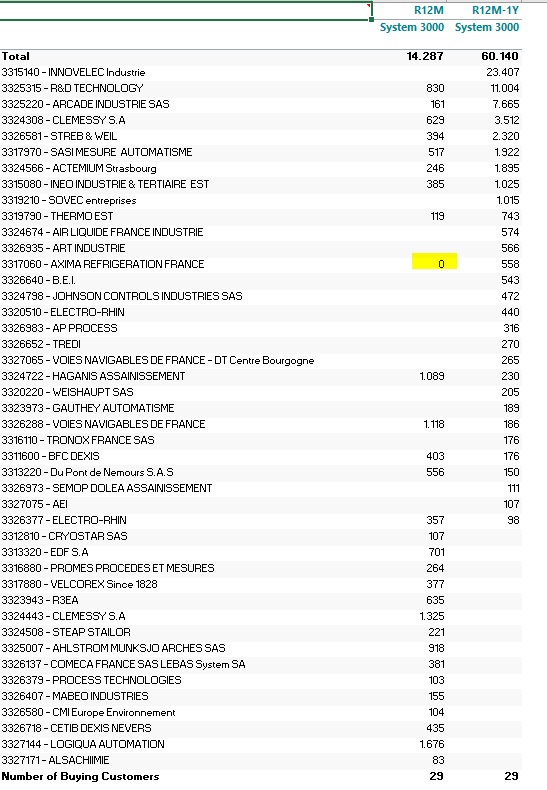• Hi Flemming,

I guess you will need to insert an extra calculation (as a new measure, m3):

• if sum(0, 0, m1) = 0 then 0 else 1

Then you Number of Buying Customers can be calculated as:

• sum(0, all, m3)

BR / Ole

• Hi Ole

Of Course - why coundn't I see that myself ;-)

Thanks

• Hi there,

I have a KPI that I want to hide, if the value it is supposed to show is empty or shows an error. I tried the trick, but eventhough the value is hidden and the option "hide if empty" is marked, the KPI is still showing. Is there another workaround?